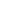# Understand the meaning of the equal sign by pairing equivalent expressions and constructing true number sentences.

EngageNY 60 min(s)Support for English Language Learners
In this lesson, students use linking cubes to write expressions and to demonstrate that they are equivalent by using the equal sign. For example, they divide one group of 5 into 3 and 2, and another group of 5 into 4 and 1, and write the equation 3 + 2 = 4 + 1.